# CircHiC tutorial¶

## Introduction¶

CircHiC is a plotting library developped for bacterial Hi-C data. It is built upon Matplotlib, the single most used Python package for 2D-graphics.

This tutorial is heavily inspired by the excellent Matplotlib tutorial written by Nicolas Rougier.

## A simple CircHic plot¶

In this section, we are going to plot data from E. coli from Lioy et al. (2018) Cell, 172(4), 771–783. The data is provided as a sample dataset of circhic. We will start with the default setting and enrich the figure step by step to make it nicer and supplement the Hi-C contact map with genomic information.

The first step is to load the data and the modules we will be using:

import matplotlib.pyplot as plt
import circhic

counts = data["counts"]
nbins = data["nbins"]


Several datasets are included in circhic, including contact maps from E. coli, B. subtilis, a chromosome from the Human cell line KBM7, etc. All of those datasets are accessible from the module circhic.datasets.

counts is a NumPy ndarray of shape (469, 469).

Before attempting any visualization, we will normalize the data using iced.

from iced.normalization import ICE_normalization
counts = ICE_normalization(counts)


### Using the defaults¶

circhic comes with a set of default settings that are built upons Matplotlib. These settings allow to customize almost any kind of properties: figure size and dpi, line width, color and style, axes, axis and grid properties, text and font properties and so on. While matplotlib defaults are rather good in most cases, you may want to modify some properties for specific cases.

circhic also requires to know more about the data plotted than Matplotlib. In particular, the library requires to know the number of bins of the Hi-C contact map. Let us instantiate a circhic figure by providing the number of bins per chromosomes to the figure. You can also provide the exact length in base pair.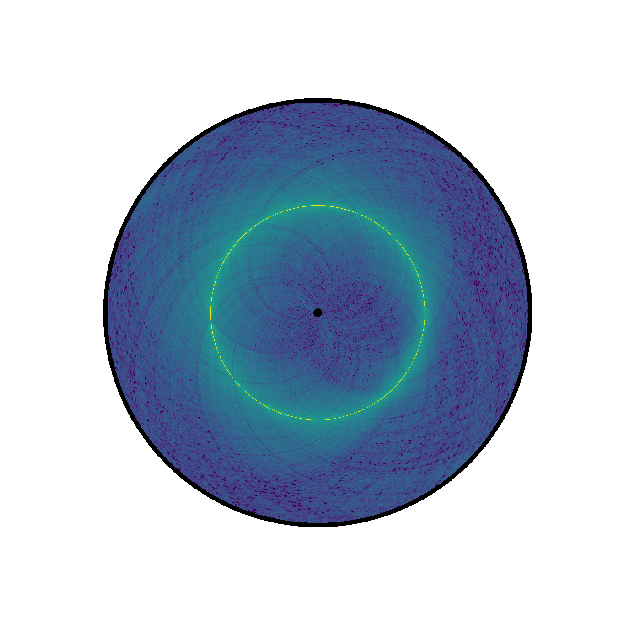counts = data["counts"]
nbins = data["nbins"]

# Now instantiate the circhic Figure
circhicfig = circhic.CircHiCFigure(lengths=nbins)
circhicfig.plot_hic(counts)


### Changing colormap and border width¶

In the script below, we have changed the default colormap used as well as the border thickness.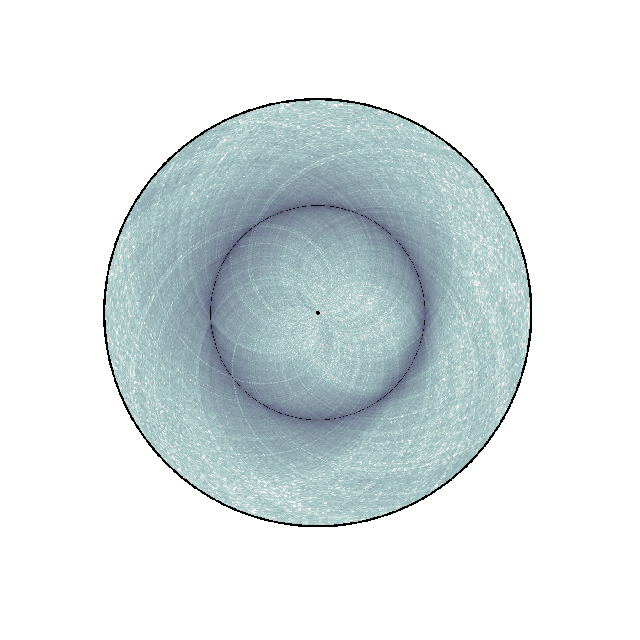nbins = data["nbins"]

# Now instantiate the circhic Figure
circhicfig = circhic.CircHiCFigure(lengths=nbins)
circhicfig.plot_hic(counts, cmap="bone_r", border_thickness=0.01)


We are now going to add a colorbar to the plot. In order to do this, we need to retrieve the mappable, ie the image, that sets the range of values. The colorbar can either be horizontal or vertical (the default).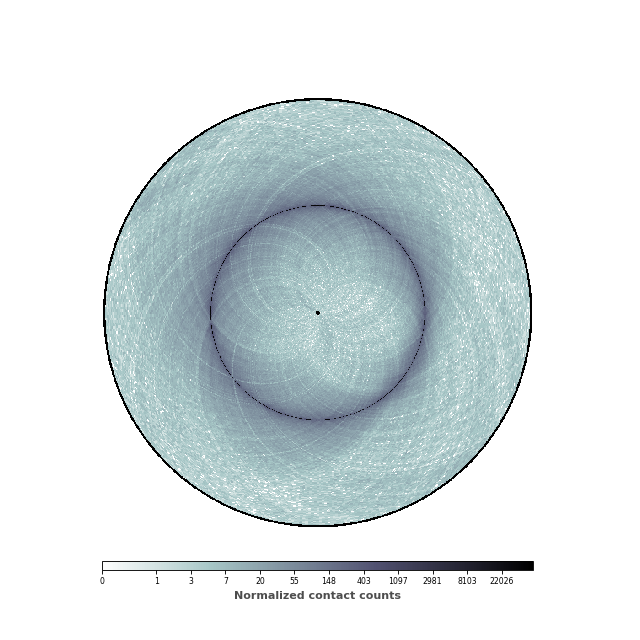nbins = data["nbins"]

# Now instantiate the circhic Figure
circhicfig = circhic.CircHiCFigure(lengths=nbins)
im, ax = circhicfig.plot_hic(counts, cmap="bone_r", border_thickness=0.01)

# Add the colorbar as a vertical colorbar
cab = circhicfig.set_colorbar(im, orientation="horizontal")
cab.set_label("Normalized contact counts", fontweight="bold", color="0.3")


Working with Matplotlib colorbar

Experienced Matplotlib users can recognized that the object returned by the CircHiCFig.set_colorbar function is a Matplotlib colorbar. As such, one can use any Matplotlib strategy to change the ticks, ticklabels, and labels of the colorbar.

### Changing the range of the colormap¶

We are now going to set the minimal and maximum value of the colorbar, in order to highlight the patterns of the contact map.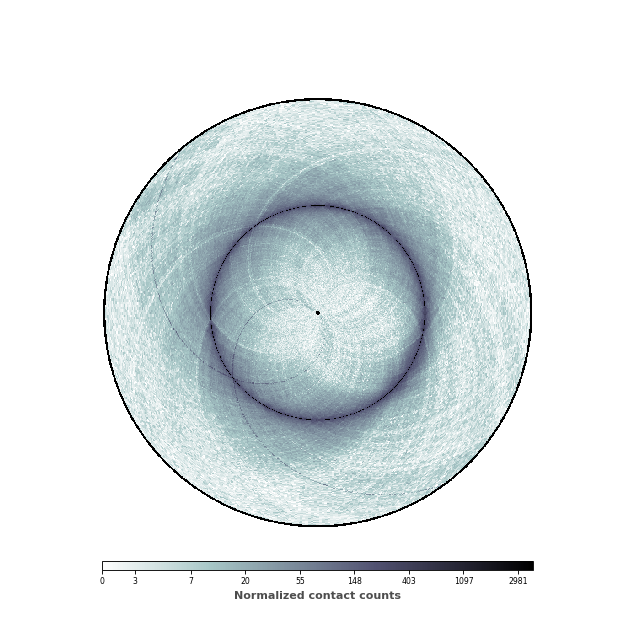data = circhic.datasets.load_ecoli()
counts = data["counts"]
nbins = data["nbins"]

# Normalize the data using ICE, and keep the biases
counts, bias = ICE_normalization(counts, output_bias=True)

# Now instantiate the circhic Figure
circhicfig = circhic.CircHiCFigure(lengths=nbins)

# Compute the extreme values
vmax = np.max([counts[i, (i+1) % counts.shape]
for i in range(counts.shape)])
vmin = np.min(counts[counts > 0]) * 10

im, ax = circhicfig.plot_hic(counts, cmap="bone_r", border_thickness=0.01,
vmin=vmin, vmax=vmax)

# Add the colorbar as a vertical colorbar
cab = circhicfig.set_colorbar(im, orientation="horizontal")
cab.set_label("Normalized contact counts", fontweight="bold", color="0.3")


### Setting the range of genomic distances plotted¶

In this figure, we would like to highlight two elements: (1) the chromosomal interaction domains (CID) (closely related to the topological associated domains in mammifers); (2) the second diagonal highlighting the enriched interactions between the two arms of the chromosome. We are thus going to adjust the range of the genomic distance plotted. To highlight the chromosomal interaction domains, we will plot only the contact counts close to the diagonal. To highlight the second diagonal, we will plot the whole range of contact count data. To facilitate readability, we will also set the inner radius to a non-zero value, in order to create a “donut” shape.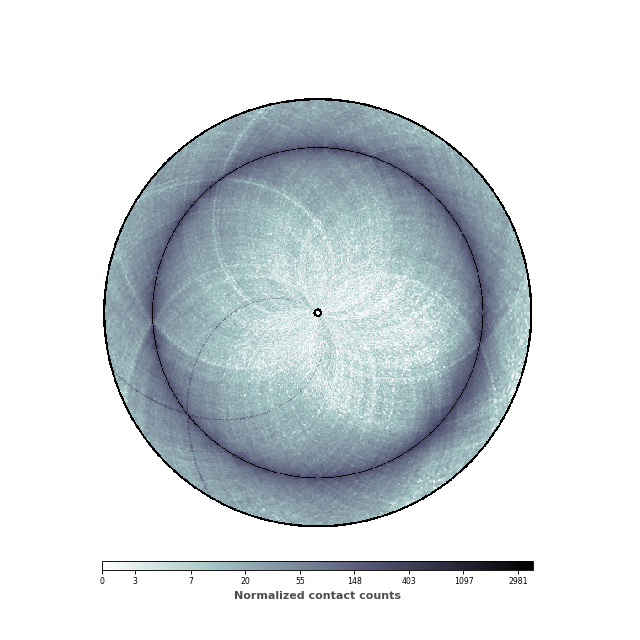inner_gdis = 200
outer_gdis = 60

im, ax = circhicfig.plot_hic(counts, cmap="bone_r", border_thickness=0.01,
inner_gdis=inner_gdis, outer_gdis=outer_gdis)


### Adding ticks and tick labels¶

Now that the contact map displays the two features we are interested in, it is time to add ticks and tick labels to the plot.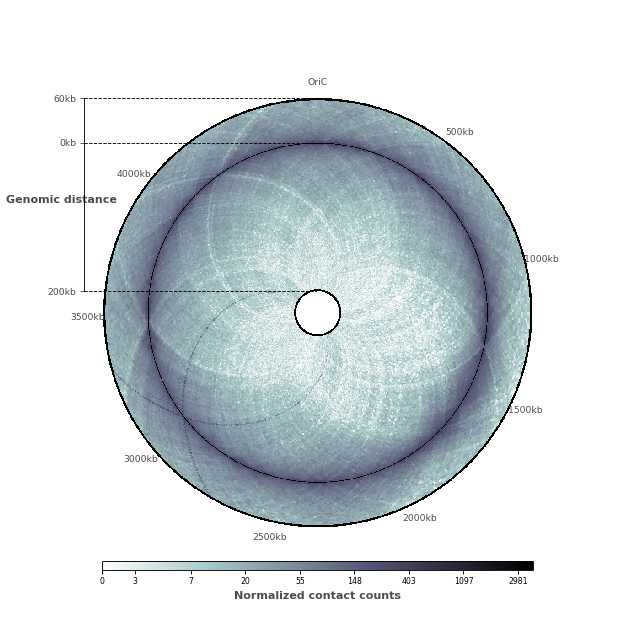And here is the entire code to reproduce this plot!

import circhic
import numpy as np

from iced.normalization import ICE_normalization

counts = data["counts"]
nbins = data["nbins"]

# Normalize the data using ICE, and keep the biases
counts, bias = ICE_normalization(counts, output_bias=True)

# Now instantiate the circhic Figure
circhicfig = circhic.CircHiCFigure(lengths=nbins)

# Compute the extreme values
vmax = np.max([counts[i, (i+1) % counts.shape]
for i in range(counts.shape)])
vmin = np.min(counts[counts > 0]) * 10

# define the inner genomid distances and the outer genomic distance plotted
inner_gdis, outer_gdis = 200, 60

im, ax = circhicfig.plot_hic(counts, cmap="bone_r", border_thickness=0.01,
inner_gdis=inner_gdis, outer_gdis=outer_gdis)

# Add the colorbar as a vertical colorbar
cab = circhicfig.set_colorbar(im, orientation="horizontal")
cab.set_label("Normalized contact counts", fontweight="bold", color="0.3")

# Now, let us add the ticks and tick labels
ticklabels = ["%dkb" % (i * 500) for i in range(9)]
tickpositions = [int(i*50) for i in range(9)]
ticklabels = "OriC"
ax = circhicfig.set_genomic_ticklabels(
tickpositions=tickpositions,
ticklabels=ticklabels,